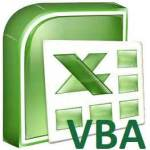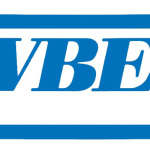# Function, Sub, Method, Property DifferencesThis tutorial explains differences among Function, Sub, Method, Property Excel / Access VBA. You may also want to read: Difference between Dim and Set Excel VBA Option Explicit to force declaration of variables Difference between ByRef ByVal Function, Sub, Method, Property Differences In my blog, I have categorized different VBA topics by their properties. If you don't understand Function, Sub, Method, Property Differences, you will find my tips confusing and difficult to use. Thos...
More

# Excel Find FunctionThis Excel tutorial explains how to use Excel FIND Function for Excel worksheet, and also explains the differences between INSTR and SEARCH function. You may also want to read: Extract text in brackets Remove text in brackets Excel Find Function Excel Find function is used to search a substring (part of the string) within a string and return the position of the first occurrence, note that Excel Find function is case sensitive. Excel Search function is very similar to Find functi...
More

# Tips on Microsoft Office Specialist for Excel exam MOSWhat is Microsoft Office Specialist for Excel exam? Microsoft Office Specialist (also known as MOS) is a certificate that can be earned if you pass an exam. Microsoft Office Specialist has exams for different Office products, which include Microsoft Word, Powerpoint, Excel, Access, Outlook, SharePoint, OneNote. Microsoft Word and Excel are slightly different, each of them have two levels, one is Core level and the other is Expert level. If you take a specific combination of exams, you can...
More

# Excel AutoCorrect OptionExcel AutoCorrect Excel AutoCorrect literally means to correct a misspell text to a correct text automatically, but you can also make use of this function to make a mapping list, to convert a text to another text automatically, so Excel AutoCorrect is more than just "Correction". Some people use it to convert abbreviation, convert language. For example, if your mapping list maps M as Mother, as you type "M" in a Cell, it will convert to "Mother". Other Office products also have the exact ...
More

# Excel iterative calculation and circular referenceExcel iterative calculation and circular reference To understand what is Excel iterative calculation, you should first understand what is circular reference. Circular reference happens when you type a formula in one Cell which refers to another Cell, but that Cell also refers back to the Cell. For example, Cell A1:  = 1+A2 Cell A2: = 1+A1 The above formula refer to each other, this is called circular reference (refer to each other forming a circle). The formula will fail and the be...
More

# Excel SUMIFS FunctionWhat is Excel SUMIFS Function? Excel SUMIF Function sum up an array (a range of values) if condition is met. Excel SUMIFS function is an advanced version of SUMIF, allow you to set multiple conditions. If you only need to apply one criteria, please read the use of SUMIF Excel SUMIF Function Syntax of Excel SUMIFS Function SUMIFS(sum_range, criteria_range1, criteria1, [criteria_range2, criteria2, ... criteria_range_n, criteria_n] ) sum_range The actual cells to add, if you wa...
More

# Excel SUMIF FunctionWhat is Excel SUMIF Function? Excel SUMIF Function sum up an array (a range of values) if condition is met. Note that you can set only one condition, for multiple conditions you need the SUMIFS Function (with "s" in suffix) Read the below article about SUMIFS Function Excel SUMIFS Function Syntax of Excel SUMIF Function SUMIF( range, criteria, [sum_range] ) range The range of cells that you want evaluated by criteria. Cells in each range must be numbers or names, arrays, or refe...
More

# Writing your first Excel Macro by recording MacroThis Excel tutorial guides you to create your first Excel Macro by Record Macro function, and explain how to modify Macro in Visual Basic Editor You may also want to read: Create Excel Add-in and add in Ribbon Excel Record Macro Writing your first Excel Macro by recording Macro, why? First of all, you should face the fact that you cannot know everything. You can use Microsoft Windows does not mean you can write your own Windows. Even if you are a Windows Administrator, it only means...
More

# Excel VBA Union MethodExcel VBA Union Method for Range Union Method returns a Range which is combined from two or more Range. In the above diagram, Union A and B returns area A plus B, where the intersection area only counts once. The first time I used Union was that I needed to do several formatting for several columnS but they were not side by side. For example, I need to change color for column A, column C and column Z,  the best way to do is to combine all three columns  as a new Range, and then apply c...
More

# VBA Excel Worksheet.Change EventThis Excel VBA tutorial explains how to use Worksheet.Change Event. You may also want to read: Excel VBA get value before Worksheet_Change event Excel automatically refresh pivot table VBA Excel Worksheet_Change Event Excel predefines some popular actions that  you would do on different Objects (worksheet, workbook, button, etc), those actions are called Event. For example, activating a worksheet is an Event, closing a workbook is an Event, clicking on a button is an event. Each Objec...
More